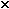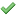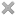User Name Remember Me? Password

 Electricity and Magnetism Electricity and Magnetism Physics Help ForumSep 10th 2010, 09:09 AM #1 Junior Member   Join Date: Feb 2010 Posts: 6 Parallel plate capacitor Here is the question... A parallel plate capacitor with paper between the plates has an electric field of 9.31104 V/m between the plates. The plates are 1.75 mm apart and the charge on each plate is 0.775 µC. Find the capacitance of this capacitor. 1F Find the area of each plate. 2m2 OKay, so the capacitance was easy to find, Q=CV, solve for C, however, I'm having a difficult time with the area of each plate. Since we know the capacitance is 4.75e-9, we can use the equation for Capacitance to find area, right? C= [(dielectric constant)x(8.85e-12)x(area)]/d rearrange: Area= (C x d)/[(dielectric constant)x8.85e-12] The dielectric constant of paper should be between 1.5 and 3, as far as I have found out, but when I use either 1.5 or 3, it doesn't work. Am I doing this right? What am I doing wrong here? Thanks in advance!!Sep 10th 2010, 09:11 AM #2 Junior Member   Join Date: Feb 2010 Posts: 6 Sorry, the question didn't copy right, but the answer to the first part war 4.75e-9 and the answer I got to the second was 0.62Sep 10th 2010, 09:15 PM #3 Senior Member   Join Date: Apr 2008 Location: HK Posts: 886 For calculating the capacitence, you should use E=Vd and Q=CV where E is the electric field strength V is the potential difference between the plates C is the capacitence Q is the charges on plate d is the separation between the plates Then you get E/d=V and V=Q/C so E/d=Q/C __________________ Good results were achieved and the new task is to become a good doctor.Tags capacitance, capacitor, dielectric, parallel, parallel plate, plateThread ToolsShow Printable VersionEmail this Page Display ModesLinear ModeSwitch to Hybrid ModeSwitch to Threaded ModeSimilar Physics Forum Discussions Thread Thread Starter Forum Replies Last Post Skyrim Electricity and Magnetism 1 Apr 28th 2011 02:50 PM prodgod Electricity and Magnetism 0 Feb 25th 2011 09:44 AM Power-Inside Electricity and Magnetism 1 Feb 8th 2011 11:11 AM mikewhant Electricity and Magnetism 13 Jan 25th 2010 10:33 AM asi123 Advanced Electricity and Magnetism 1 Feb 13th 2009 07:52 PM